# A novel vehicle feature extraction algorithm based on wavelet moment

A novel vehicle feature extraction algorithm based on wavelet moment

Xiaoru Song Song Gao Chaobo Chen

College of electronic information engineering, Xi'an Technological University, Xi'an 710021, China

Corresponding Author Email:
gao2223@qq.com
Page:
223-242
|
DOI:
https://doi.org/10.3166/TS.35.223-242
|
Accepted:
|
Published:
31 December 2018
| Citation

OPEN ACCESS

Abstract:

The moments of vehicle image features differ in magnitude and depend on the scale factor. To solve these problems, this paper proposes a feature extraction algorithm based on wavelet moment method. Focusing on the principles of invariant moment and wavelet energy, the proposed algorithm was applied to extract the features of pretreated images on actual vehicles. Specifically, the pretreated images were subjected to wavelet decomposition, yielding tertiary sub-images. Then, the sub-images were processed by taking the modified Hu invariant moment as the feature. The results show that the features extracted by our algorithm remained invariant after translation, rotation and scale transformation, and reflected the vital and essential attributes of the vehicle images. The recognition rate of our algorithm was 13.5% higher than that of the traditional Hu moment. The research findings shed new light on image classification and recognition.

Keywords:

feature extraction, modified hu invariant moment, wavelet moment, target recognition

1. Introduction

Vehicle identification system is an important part of intelligent transportation system. There is much great convenience to target recognition due to the extremely rich amount of information contained in the image and the image sensors are lower cost. So it is widely used in intelligent transportation systems that the image recognition based on machine vision. The image recognition mainly consists of image preprocessing, feature extraction and classification decision. The feature extraction phase is a key part of image recognition. The reliability of the feature vectors affects the recognition rate directly(Hadavi & Shafahi, 2016; Zhou et al., 2017).

Therefore, various methods for extracting vehicle features have been proposed by scholars, but each method has its own specific application scenarios with limitations (Zhu et al., 2017; Boukerche et al., 2017). More common shape feature extraction methods include Fourier descriptor, Hough transform, shape matrix and moment invariant. The Fourier descriptor has a good description of the closed curve, but it is less effective for the composite closed curve (Jia & Duan, 2017). The Hough transform is mainly used to detect parallel lines and boundary direction histograms (Zhang et al., 2015; Wafy & Madbouly, 2016), this method obviously is not suitable for non-parallel lines in the vehicle contour.

The wavelet transform transforms the extracted target image into multiple frequencies and decomposes it into two sub-images with different spatial and frequency. Image energy is mainly concentrated in the low frequency part. It reflects the overall outline of the image. The wavelet energy does not have translation, rotation, and proportional invariance. In order to ensure the stability of the extracted feature vector, it is necessary to perform secondary extraction on the obtained sub-image. The moment feature has good stability through various moments reflect the shape feature information of the object. But there still has shortcomings. Such as high-order moments are more sensitive to scale factors in two-dimensional discrete cases. And the magnitudes of the moments of the moment feature are quite different. Therefore, wavelet energy feature extraction or invariant moment feature extraction is used alone; the better feature vectors cannot be obtained. In order to solve this problem, the moment feature is modified. In the paper the modified moment feature is used to extract the sub-image twice to obtain the wavelet moment feature quantity. And the sub-image is decomposed by wavelet. The wavelet moment is used for feature extraction of vehicle images, and the vehicle's various attitude image feature quantities are compared and analyzed, it is expected that the stability of the wavelet moment feature vector is obtained, the translation, rotation, and scale invariance are satisfied, and the recognition rate of the target is improved.(Shi et al., 2016; Sridevi et al., 2017; Zhou et al., 2017; Liu & Qiang, 2017; Liu et al., 2017; Yan and Zhang, 2017)

The rest of the paper is organized as follows. The characteristics of wavelet energy problem are presented in the section 1. The part 2 is analysed the moment and invariant moment theory. The next section is the key. The section 3 is extractions of wavelet moment features. The simulation of the wavelet moment feature extraction algorithm is in the section 4. it is including the translation invariance of wavelet moments, Rotation invariance of wavelet moments and Proportional invariance of wavelet moments. The verification of vehicle identification is in the section 5. At last, conclusions are drawn.

2. Characteristics of wavelet energy

The basic principle of wavelet transform is to decompose the target image by multiple frequencies. According to different frequencies, the target image is decomposed into two sub-images with different space and frequency (Dooley et al., 2015). Liu et al. used wavelet transform to decompose the image to obtain a second-level sub-image, and the texture features of the sub-image were extracted. There are still some shortcomings to this approach:

Extracting the vehicle target image by wavelet transform is based on the frequency characteristics of the image, and the low and high frequency parts of the wavelet domain are extracted, the two parts are compared and then formed, but the energy to be obtained is distributed at the intermediate frequency level, if the characteristics of the vehicle to be extracted are mainly concentrated in the high frequency stage, the characteristics obtained by the method will affect the classification effect of the target. According to the basic principle of the above wavelet transform, the frequency decomposition operation of the image occurs on each frequency, so the calculation amount is increased, the complexity is increased, and the operation speed is lowered.

3. Analyses of Moment and Invariant Moment Theory

In 1962, the feature vector that was not changed by image translation, target rotation, and target scaling was proposed by M.K. Hu, namely invariant moment theory, and it is widely used in the field of image processing, such as vehicle detection and identification in intelligent transportation systems.

2.1. Characteristics and disadvantages of Hu invariant moments

For two-dimensional continuous functions f(x,y), (p+q) moment is defined as

$m_{p q}=\int_{-\infty}^{\infty} \int_{-\infty}^{\infty} x^{p} y^{q} f(x, y) d x d y$     (1)

the center distance is defined as：

$\mu_{p q}=\int_{-\infty}^{\infty} \int_{-\infty}^{\infty}(x-\overline{x})^{p}(y-\overline{y})^{q} f(x, y) d x d y$          (2)

where

$\overline{x}=\frac{m_{10}}{m_{00}} \quad \overline{y}=\frac{m_{01}}{m_{00}}$

a set of 7 invariant moments from second and third moments，

$\phi_{1}=\eta_{20}+\eta_{02}$

$\phi_{2}=\left(\eta_{20}-\eta_{02}\right)^{2}+4 \eta_{11}^{2}$

$\phi_{3}=\left(\eta_{30}-3 \eta_{12}\right)^{2}+\left(3 \eta_{21}-\eta_{03}\right)^{2}$

$\phi_{4}=\left(\eta_{30}+\eta_{12}\right)^{2}+\left(\eta_{21}+\eta_{03}\right)^{2}$

\begin{aligned} \phi_{5}=&\left(\eta_{30}-3 \eta_{12}\right)\left(\eta_{30}+\eta_{12}\right)\left[\left(\eta_{30}+\eta_{12}\right)^{2}-3\left(\eta_{21}+\eta_{03}\right)^{2}\right]+\left(3 \eta_{21}-\eta_{03}\right)\left(\eta_{21}+\eta_{03}\right)\left[3\left(\eta_{30}+\eta_{12}\right)^{2}-\left(\eta_{21}+\eta_{03}\right)^{2}\right] \\ \phi_{6}=&\left(\eta_{20}-\eta_{02}\right)\left[\left(\eta_{30}+\eta_{12}\right)^{2}-\left(\eta_{21}+\eta_{03}\right)^{2}+4 \eta_{11}\left(\eta_{20}+\eta_{12}\right)\left(\eta_{21}+\eta_{03}\right)\right] \end{aligned}

\begin{aligned} \phi_{1}=&\left(3 \eta_{21}-\eta_{03}\right)\left(\eta_{20}+\eta_{12}\right)\left[\left(\eta_{30}+\eta_{12}\right)^{2}-3\left(\eta_{21}+\eta_{03}\right)^{2}\right]+\left(3 \eta_{12}-\eta_{30}\right)\left(\eta_{21}+\eta_{03}\right) \\ &\left[3\left(\eta_{30}+\eta_{12}\right)^{2}-\left(\eta_{21}+\eta_{03}\right)^{2}\right] \end{aligned}

the above seven moments have characteristic stability.

In discrete state，set (m’, n’) to the target coordinate is transformed by the scale factor ρ, the original coordinates (m, n), the two of them satisfy the following relationship：

$\left\{\begin{array}{l}{x=\rho x} \\ {y^{\prime}=\rho y}\end{array}\right.$   (3)

$x^{\prime}-\overline{x}^{\prime}=\rho(x-\overline{x})$

$y^{\prime}-\overline{y^{\prime}}=\rho(y-\overline{y})$                 (4)

$\operatorname{bring}\left(x^{\prime},-\overline{x^{\prime}}\right),\left(y^{\prime},-\overline{y^{\prime}}\right)$ to $\mu^{\prime} p q, \quad$ exist

$\mu_{p q}^{\prime}=\rho^{p+q} \mu_{p q}$ (5)

normalized center distance，

$\eta_{p q}^{\prime}=\frac{\mu_{p q}^{\prime}}{\mu_{00}^{\prime \gamma}}=\frac{\rho^{p+q} \mu_{p q}}{\mu^{\gamma}_{00}}=\rho^{p+q} \eta_{p q}$     (6)

It can be seen from equation $(6)$ that the $\eta_{\text {pg }}^{\prime}$ and $\eta_{p g}$ scale factors are proportional to each other and vary with the order p+q of the moment. The above formula shows that the seven invariant moments in the Hu moment will change due to the change of the scale factor in the discrete case.

2.2. Correction of invariant moment components

The above analysis shows that the Hu moment is affected by the scale factor in the discrete state, and the wavelet transform has the frequency domain problem. According to formula (6), the relationship between $\eta_{p q}$ and $\eta_{p q}^{\prime}$ before and after the scale factor transformation can be derived for seven moments.

$\phi_{1}^{\prime}=\rho^{2} \phi_{1}$   (7)

$\phi_{2}=\rho^{4} \phi_{2}$        (8)

$\phi_{3}=\rho^{6} \phi_{3}$      (9)

$\phi_{4}^{\prime}=\rho^{6} \phi_{4}$ (10)

$\phi_{5}^{\prime}=\rho^{12} \phi_{5}$    (11)

$\phi_{6}=\rho^{8} \phi_{6}$     (12)

$\phi_{7}^{\prime}=\rho^{12} \phi_{7}$    (13)

According to the relationship of equations (7) to (13), seven new invariant moment formulas are obtained by modifying the seven moments without being affected by the scale factor, as follows

$\phi_{2}^{*}=\phi_{2}^{\prime} / \phi_{1}^{2}$   (14)

$\phi_{3}^{*}=\phi_{3}^{\prime} / \phi_{1}^{\prime 3}$   (15)

$\phi_{4}^{*}=\phi_{4}^{\prime} / \phi_{1}^{\prime 3}$   (16)

$\phi_{5}^{*}=\phi_{5} / \phi_{1}^{6}$   (17)

$\phi_{6}^{*}=\phi_{6}^{\prime} / \phi_{1}^{\prime 14}$    (18)

$\phi_{7}^{*}=\phi_{7} / \phi_{1}^{6}$      (19)

The new invariant moment does not change due to the change of the scale factor, and still maintains translation and rotation invariance.

3. Extractions of wavelet moment features

The Hu invariant moment still has problems, the above shows the expression of the common moment and center moment of the Hu moment, the extracted features of the target image to be tested should satisfy the change without changing the position of the target image, changing the pose, and scaling the image. The extracted features of the target image to be tested should satisfy the characteristics that do not change with the position change, pose change and the scaling of the image, the vehicle image feature is represented by the origin moment or the center distance, the first point is satisfied only. After the center distance is normalized, the feature moment satisfies the first point and the third point. Therefore, the two representations described above cannot meet these three requirements at the same time. In order to solve this problem, this paper introduces an invariant moment feature extraction algorithm based on wavelet transform, the method combines the advantages of the two methods on the basis of analyzing the Hu invariant moment and the lack of wavelet energy. Firstly, wavelet decomposition of the target image is performed to obtain sub-images of each frequency, and then the modified Hu moment features are extracted for these sub-images, The computational complexity of this method is effectively reduced, and the extracted image features can meet the above three requirements, this facilitates the recognition of the image.

The basic idea of the feature extraction based on wavelet variation is as followings:

- The target image to be identified is normalized to avoid that the wavelet energy feature changes as the image is scaled.

- Using wavelet decomposition, multi-level frequency sub-images of the target image are obtained.

- Calculate the energy of each sub-image using equation (20), si(x,y)indicates a sub image, where x=0,1,..,M-1; y=0,1,…,N-1;

The energy of the sub-image is

$e_{i}=\frac{1}{M N} \sum_{x=0}^{M-1} \sum_{y=0}^{N-1} s_{i}(x, y)^{2}(1 \leq i \leq k)$      (20)

Where the sub image size is M´N, after multi-scale decomposition, the number of low-frequency sub-images is k.

- after calculating the energy of each sub-image, the Hu moment feature extraction is performed on the sub-image.

- after the operation of c and d, the image feature vector based on wavelet moment is constructed.

The feature vector obtained by this method can reflect the essential features of the vehicle target image, and the three requirements described above are simultaneously satisfied

4. Verification of wavelet moment feature extraction algorithm

Using MATLAB as the simulation software, the validity of the feature vector extraction method proposed in this paper is verified, after corresponding preprocessing of the vehicle target image, the wavelet moment feature of the vehicle target image is extracted to verify the validity of the wavelet moment. To verify the validity of the method for the first condition (translation), vehicle images at the left and right positions at the same distance from the acquisition system is collected. To verify the validity of the method for the second condition (rotation), the front and side views of the vehicle are collected, at the same time ensure that the distance between the car and the acquisition system remains. To verify the validity of the method for the third condition, two vehicle images 5 meters (reference map) and 10 meters away from the acquisition system were collected and compared. In the effectiveness experiment of image wavelet moment, image pre-processing is performed on the acquired images; wavelet moment feature extraction is then performed on the processed image.

4.1. Rotation invariance of wavelet moments

The acquisition system collects the left side of the vehicle as a reference map, and the vehicle rotates 90°(front side) to study the wavelet moment rotation invariance. As shown in Fig. 1 and Fig. 2, Fig. 1 is a reference image, a target left image, and Fig. 2 is a rotated 90°image.

## f1.jpgFigure 1. Vehicle reference image

## f2.jpg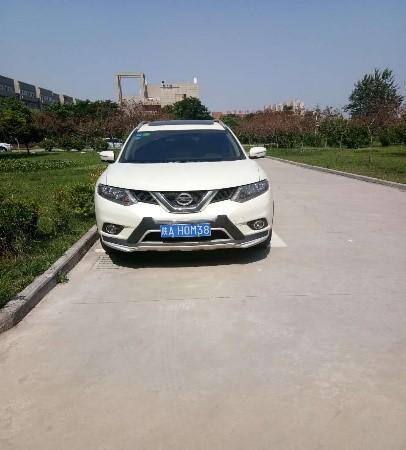Figure 2. Vehicle front side image

After the image is grayed out, median filtered, canny edge extraction, Hough transform, image segmentation preprocessing, wavelet moment features are extracted. The pre-processed images are shown in Fig.3 and Fig.4. Fig.3 shows the vehicle reference image. Fig.4 shows the vehicle front side edge image rotated 90°.

## f3.pngFigure 3. Side edge

## f4.pngFigure 4. Front edge image

The low-frequency image of the three-order wavelet variation obtained by wavelet decomposition is shown in Fig.5 and Fig.6., Fig. 5 is a three-level wavelet decomposition of the target front image, and Fig. 6 is a three-level wavelet decomposition of the rotated 90°image.

## f5.png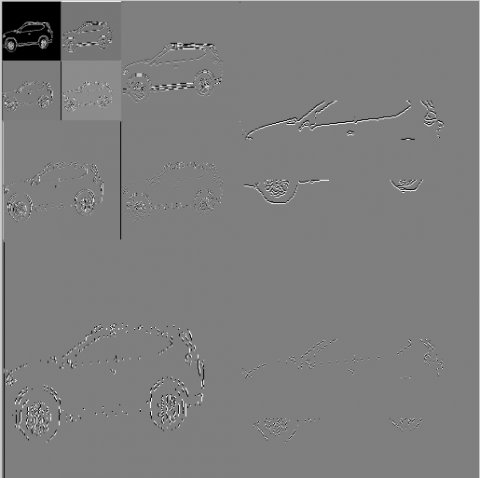Figure 5. Side Wavelet Decomposition

## f6.png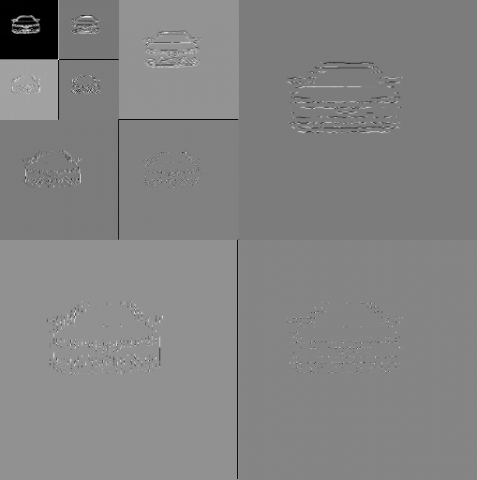Figure 6. Frontal wavelet decomposition

Perform three-level wavelet decomposition on the reference image and the rotated 90° target image to obtain a three-level sub-image, then, the quadratic modified Hu moment feature extraction is performed on each sub-image, and the tables 1 and 2 are obtained, Table 1 shows the three-level wavelet moment feature vector of the reference image, table 2 shows the three-order wavelet moment feature vector of the rotated 90°target image. As shown in Table 1 and Table 2.

Table 1. Three-level wavelet moment feature vector of reference image

 Energy Level (e+07) Feature Vector $\phi_{1}^{*}$ $\phi_{2}^{*}$ $\phi_{3}^{*}$ $\phi_{4}^{*}$ $\phi_{5}^{*}$ $\phi_{6}^{*}$ $\phi_{7}^{*}$ First level e1 4.0623 5.1694 13.7464 18.2123 17.8795 39.4207 25.2990 37.2896 Second level e2 4.0373 5.8618 15.1127 20.3102 19.9578 43.6897 28.0673 41.5301 Third level e3 3.9881 6.5570 16.4432 22.4757 22.0707 48.0406 30.8578 45.9324

Table 2. Rotate 90° third-order wavelet moment vectors

 Energy Level (e+07) Feature Vector $\phi_{1}^{*}$ $\phi_{2}^{*}$ $\phi_{3}^{*}$ $\phi_{4}^{*}$ $\phi_{5}^{*}$ $\phi_{6}^{*}$ $\phi_{7}^{*}$ First level e1 1.1567 5.6989 16.9058 19.8357 17.7344 37.5237 28.9471 36.5525 Second level e2 1.1485 6.3915 18.1603 21.8288 19.8187 41.6584 31.6319 40.6749 Third level e3 1.1325 7.0824 19.3498 23.7427 21.9089 45.7723 34.4043 44.7643

The preprocessed image is first subjected to three-level wavelet decomposition to obtain a three-level sub-image, and the energy of each sub-graph is obtained. It can be seen from Table 1 and Table 2, for the same target image, the energy of each sub-image is approximately equal。Finally, the second wavelet moment feature vector extraction is performed on the sub-image, the seven wavelet moment vector values corresponding to the sub-images of the two target images are compared respectively, the seven vectors are in the range of 2%, and the values are equal. Therefore, the wavelet moment proposed in this paper has rotation invariance.

4.2. Translation invariance of wavelet moments

Using the target and the image acquisition system to make a specific positional relationship, respectively using the left and right sides of the same distance from the image acquisition system to simulate the translation of the target image, the right shift image is preprocessed firstly, and the target right shift edge detection image is obtained as shown in fig.7, Fig.8 is a three-level wavelet decomposition diagram of the right-shifted image, as shown in Fig.8.The second modified Hu moment feature extraction is performed on the right-shifted three-level sub-image, and the wavelet moment feature vector of each sub-image is obtained, as shown in Table 3.

## f7.pngFigure 7. Target right shift edge image

## f8.pngFigure 8. Three-level wavelet decomposition of the right-shifted image

Similarly, the pre-processed image is firstly decomposed by three-level wavelet, and the second wavelet moment feature vector is extracted for each sub-image to obtain seven invariant moment components per level, It can be seen from Table 1 and Table 3, the right-shift target image wavelet feature vector and the reference image are numerically compared in a 2% error range, The wavelet moment feature vector of the right-shifted image is approximately equal to the wavelet moment feature vector of the reference image, so the wavelet moment proposed in this paper has translation invariance.

Table 3. Three-dimensional wavelet moment feature vector of right shift image

 Energy Level (e+07) Feature Vector $\phi_{1}^{*}$ $\phi_{2}^{*}$ $\phi_{3}^{*}$ $\phi_{4}^{*}$ $\phi_{5}^{*}$ $\phi_{6}^{*}$ $\phi_{7}^{*}$ First level e1 3.7024 5.2431 12.6626 18.3211 20.1388 40.8018 26.5110 39.9802 Second level e2 5.0120 5.9724 14.0236 20.0273 22.3524 43.8201 30.1278 44.5484 Third level e3 38.2051 6.7852 15.4547 21.9559 24.3622 48.3251 33.0875 47.6276

4.3. Proportional invariance of wavelet moments

The images of 5 meters and 10 meters away from the image acquisition system (reference map) were used to simulate the scaling of the image to study the proportional invariance of the wavelet moment，Fig.9 is a target image of the target image acquisition system 10 meters, and Fig.10 is a three-level wavelet decomposition diagram of the 10 meter image, as shown in Fig.9 and Fig.10.

## f9.png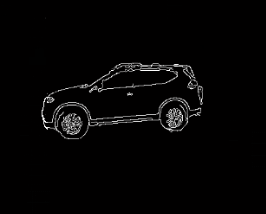Figure 9. Image edge map of 10m image

## f10.png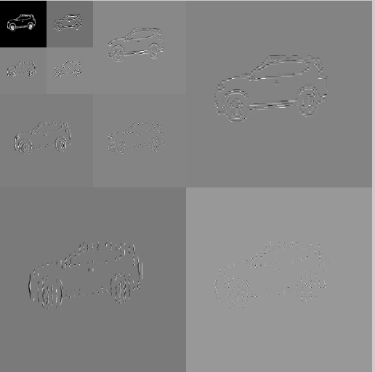Figure 10. Three-level wavelet decomposition of 10m image

The second-order Hu-moment feature extraction is performed on the 10-meter three-level sub-image to obtain the wavelet moment feature vector of each sub-image. The details are shown in Table 4.

Table 4. Three-dimensional wavelet moment feature vector of 10m image

 Energy Level (e+07) Feature Vector $\phi_{1}^{*}$ $\phi_{2}^{*}$ $\phi_{3}^{*}$ $\phi_{4}^{*}$ $\phi_{5}^{*}$ $\phi_{6}^{*}$ $\phi_{7}^{*}$ First level e1 6.7281 5.4150 11.8441 18.7526 21.9409 43.4991 27.8764 44.3121 Second level e2 6.6769 6.1079 13.2306 20.8301 24.0254 47.6703 30.6548 48.5056 Third level e3 6.5764 6.8005 14.6164 22.9102 26.1006 51.8419 33.4245 52.7565

Similarly, the pre-processed image is firstly decomposed by three-level wavelet, and the second wavelet moment feature vector is extracted for each sub-image, and seven invariant moment components of each level are obtained. It can be seen from Table 1 and Table 4, the wavelet feature vector of the 10m target image compared with the reference image are in the 2% error range, and the 10 m image wavelet moment feature vector is approximately equal to the reference image wavelet moment feature vector. Therefore, the wavelet moment proposed in this paper satisfies the translation invariance.

Compare the target's seven invariant moment values at each level after translation, rotation, and scaling, the seven wavelet moment feature vectors in the first-order energy are shown in Fig.11, the seven wavelet moment feature vectors in the second-order energy are shown in Fig.12, the seven wavelet moment feature vectors in the third-order energy are shown in Fig.13.By the target translation, rotation, and scaling, the values of the seven wavelet moment feature vectors in each level are approximately equal. Therefore, the wavelet moment has translation, rotation, and scale invariance, it can reflect the characteristics of the image.

## f11.png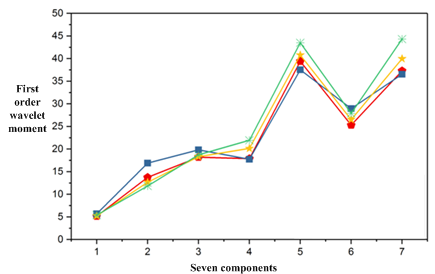Figure 11. First-order wavelet moment feature vecto

## f12.pngFigure 12. Second-order wavelet moment feature vector

## f13.pngFigure 13. Third-order wavelet moment feature vector

It can be seen from Fig.11, Fig.12 and Fig.13 that the seven wavelet moment feature vector curves on the primary, secondary and tertiary sub-images are consistent in four cases. Therefore, the wavelet moment has translation, rotation, and scale invariance. It can reflect the characteristics of the image and provide feature data for the target recognition.

5. Vehicle identification

5.1. Minimum neighbor distance

The nearest neighbor method measures the degree of similarity between the test vector and the sample vector. Using the minimum distance to measure this similarity, the Euclidean distance between the test vector and the sample vector is obtained. If the value of Euclidean distance is larger, it indicates that the test vector is different from the template sample. If the value is smaller, it can be regarded as the same type.

The Euclidean distance between xi and xj is defined as:

$d\left(x_{i}, x_{j}\right)=\sqrt{\sum_{k=1}^{n}\left(x_{i}(k)-x_{j}(k)\right)^{2}}$     (21)

After the Euclidean distance between the samples is obtained, the minimum value is set according to the Euclidean distance between the tested sample and the known sample, set with p class, {wi, i=1,2,…p}, Similar sample template is {Ni, i=1,2,…n}, the discriminant function for discriminating which class the unknown sample belongs to is：

$g_{i}(x)=\min _{k}\left\|x-x_{i}^{k}\right\|, \quad k=1,2, \ldots, N_{i}$       (22)

Decision rule:

If satisfied:

$g_{i}(x)=\min _{i}\left\{g_{i}(x)\right\}, \quad i=1,2, \ldots, p$        (23)

Then the decision is: xÎwj

5.2. Vehicle identification experiment

The actual vehicle is subjected to a simulation experiment, the specific steps are as follows:

(1) Use the image acquisition system, the image of the vehicle to be identified is obtained.

(2) Use the above analysis theory, the two types of images are separately subjected to image preprocessing, geometric feature vector extraction, and wavelet moment feature vector.；

(3) Calculate the average of the feature quantities of the same sample, and the feature library is established.；

(4) Calculate the European distance：

$\sigma=\sqrt{\left(X_{1}-X_{i 1}\right)^{2}+\left(X_{2}-X_{i 2}\right)^{2}+\ldots+\left(X_{n}-X_{i n}\right)^{2}}$         (24)

In equation (24), Xi is the characteristic value of the sample to be tested, σ and the threshold are compared.

(5) According to the comparison result, when the threshold is less than the threshold, the sample to be tested and the template are classified into one class, and if it is greater than or equal to the threshold, it is judged as “interference”.

(6) Take the target of “interference” as a sample, the eigenvalues of such targets are recalculated together with the previous samples of the same type, the new mean of the calculated sample is written into the signature database of the sample as a new criterion

The experimental samples are: car, truck, electric motorcycle. The visible light images of the four front and rear left and right views of the car are collected, as shown in Fig.14, the visible light images of the four front and rear left and right sides of the truck are collected, as shown in Fig.15, the front and rear visible light images of the electric motorcycle are collected, as shown in Fig.16.

## f14.pngFigure 14. Car position pictures in all directions

## f15.jpgFigure 15. Truck position pictures in all directions

## f16.png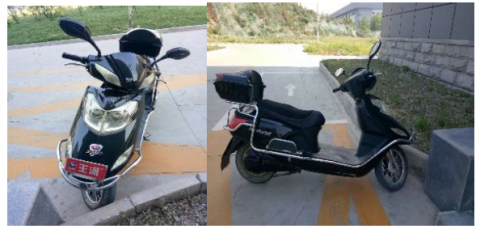Figure 16. Electric motorcycle position pictures in all directions

In the experiment, the visible light camera was adopted as a single sensor, and there are three kinds of target samples, namely: a certain type of car, truck, electric motorcycle, the three types of targets are in a specific positional relationship with the acquisition system, and the original visible light image is obtained. After translation, rotation and scaling, 20 images of each type of target are obtained, among them, 1-4 are obtained by panning the original image, 5-16 are rotated by the original image, and 17-20 are obtained by scaling the original image. There are 60 kinds of visible light images in three types. The sample images are preprocessed, the Hu moment and wavelet moment of the sample image are extracted, and the feature library is established separately. Take 10 visible images of the car as a test set, the car sensor recognition experiment was carried out，in the same way, the image of the truck and the electric vehicle was taken, and the sensor identification experiment was carried out, the Euclidean distance between the feature vector extracted from the test set and the feature vector in the template library is calculated, and the minimum value in the distance is obtained, that is, the vehicle corresponding to the minimum value is the recognition result. The visible light images are collected, and the Hu moment and wavelet moment feature vectors are extracted respectively. The recognition results in the two cases are obtained by the minimum distance method, as shown in Tables 5 and 6.

Table 5. Hu moment is used for the recognition rate of vehicle images

 Category Car Truck Electric bicycle Training set G1(50) Samples Number Samples Number Samples Number Test set G2(20) Right Wrong Right Wrong Right Wrong 4 6 3 7 3 7 Recognition rate (%) 40 30 30

Table 6. Invariant moments for the recognition rate of vehicle images

 Training set G1(50) Samples Number Samples Number Samples Number Test set G2(20) Right Wrong Right Wrong Right Wrong 5 5 5 5 6 4 Recognition rate (%) 50 50 60

Vehicle identification is carried out for car, truck and electric motorcycle respectively. Image preprocessing is performed on the acquired images. Hu moments and wavelet moment features of the target images are extracted respectively. The minimum adjacent distance classification method is used to identify cars, trucks and electric vehicles. Table 5 shows that the Hu moments of the three vehicles are feature vectors, and the car recognition rate is 40%, the truck recognition rate is 30%, and the electric vehicle recognition rate is 30%. Table 6 shows that the wavelet moments of the three vehicles are extracted as feature vectors; they are 50% for the car, 50% for the truck, and 60% for the electric vehicle. Comparing Table 5 and Table 6 for the recognition rate of various vehicles, the conclusion can be drawn: for the same target image, the two feature extraction methods are compared, and the wavelet moment of the image is extracted, and the final recognition result is better. Therefore, based on the wavelet moment feature extraction method, the vehicle image classification result is well obtained.

6. Conclusion

In this paper, the characteristics of wavelet energy and Hu invariant moment are introduced in detail. On the basis of the influence of the scale factor on Hu moment, the seven components of Hu moment are modified. A feature extraction method based on wavelet moment is proposed and applied to vehicle feature extraction. Experiments show that the feature quantity obtained by this method has stability and is not sensitive to the translation, rotation and scaling of the target. Through the vehicle recognition experiment, the Hu moment and the wavelet moment of the image are respectively extracted to obtain the respective recognition results; the experimental results show that the recognition rate of the wavelet moment of the extracted image is higher than that of the traditional Hu moment feature extraction. The wavelet moment feature quantity proposed in this paper can reflect the important and original attributes of the image and provide feature vectors for subsequent vehicle identification.

Acknowledgment

This project is grateful for the following projects: National Key R&D Program (2016YFE0111900), Shaanxi International Science and Technology Cooperation Program (2018KW-022 and 2017KW-009), Shaanxi Natural Science Foundation (2018JQ5009 and 18JK0398), and the independent intelligent control research and innovation team support this topic.

References

Boukerche A., Siddiqui A. J., Mammeri A. (2017). Automated Vehicle Detection and Classification. ACM Computing Surveys, Vol. 50, No. 5, pp. 1-39. https://doi.org/ 10.1145/3107614

Dooley D., Mcginley B., Hughes C., Kilmartin L. (2015). A Blind-Zone Detection Method Using a Rear-Mounted Fisheye Camera With Combination of Vehicle Detection Methods. IEEE Transactions on Intelligent Transportation Systems, pp. 1-15. https://doi.org/ 10.1109/TITS.2015.2467357

Hadavi M., Shafahi Y. (2016). Vehicle identification sensor models for origin–destination estimation. Transportation Research Part B Methodological, Vol. 89, pp. 82-106. https://doi.org/ 10.1016/j.trb.2016.03.011

Jia J., Duan H. (2017). Automatic target recognition system for unmanned aerial vehicle via back propagation artificial neural network. Aircraft Engineering and Aerospace Technology, Vol. 89, No. 1, pp. 145-154. https://doi.org/ 10.1108/AEAT-07-2015-0171

Liu B., Qiang G. (2017). Moment Invariants Based on Biorthogonal Wavelet Transform. Acta Electronica Sinica, Vol. 45, No. 4, pp. 826-831. https://doi.org/ 10.3969/j.issn.0372-2112.2017.04.009

Liu X., Li C., Tian L. (2017). Hand Gesture Recognition Based on Wavelet Invariant Moments. Proceedings - 2017 IEEE International Symposium on Multimedia, pp. 459-464. https://doi.org/ 10.1109/ISM.2017.91

Shi Q., Abdel-Aty M., Yu R. (2016). Multi-level Bayesian safety analysis with unprocessed Automatic Vehicle Identification data for an urban expressway. Accident Analysis & Prevention, Vol. 88, pp.68-76. https://doi.org/ 10.1016/j.aap.2015.12.007

Sridevi T., Swapna P., Harinath K. (2017). Vehicle Identification Based on the Model, IEEE, International Advance Computing Conference. pp. 566-571. https://doi.org/ 10.1109/IACC.2017.0122

Wafy M., Madbouly A. (2016). An Efficient Method for Vehicle License Plate Identification Based on Learning a Morphological Feature. Jet Intelligent Transport Systems, Vol. 10, No. 6, pp. 389-395. https://doi.org/ 10.1049/iet-its.2015.0064

Yan Q., Zhang X. H. (2017). Image Denoising Method Based on Wavelet Transform and Bilateral Filter in Vehicle Gesture Recognition. Transactions of Beijing Institute of Technology, Vol. 37, No. 4, pp. 376-380. https://doi.org/ 10.15918/j.tbit1001-0645.2017.04.009

Zhang F. Y., Chen R. B., Li Y., Guo X. C. (2015). Detection of broken manhole cover using improved Hough and image contrast. Journal .of Southeast University, Vol. 31, No. 4, pp. 553-558.

Zhou Q., Liu H., Hu X., Deng B. F., Zhang H. B., Hu H., Zuo D., Xu J. Y. (2017). The application of relative moment and wavelet moment in target obstacle recognition, IEEE International Conference on Information and Automation. pp. 1703-1707 https://doi.org/ 10.1109/ICInfA.2016.7832092

Zhou Y., Liu L., Shao L. (2017). Vehicle Re-Identification by Deep Hidden Multi-View Inference. IEEE Transactions on Image Processing, Vol. 27, No. 7, pp. 3275-3287. https://doi.org/ 10.1109/TIP.2018.2819820

Zhu J. Q., Zeng H. Q., Du Y. Z., Lei Z., Zheng L. X., Cai C. H. (2017). Joint Feature and Similarity Deep Learning for Vehicle Re-identification. IEEE Access, Vol. 99, No. 1, pp. 1-19. 10.1109/ACCESS.2018.2862382